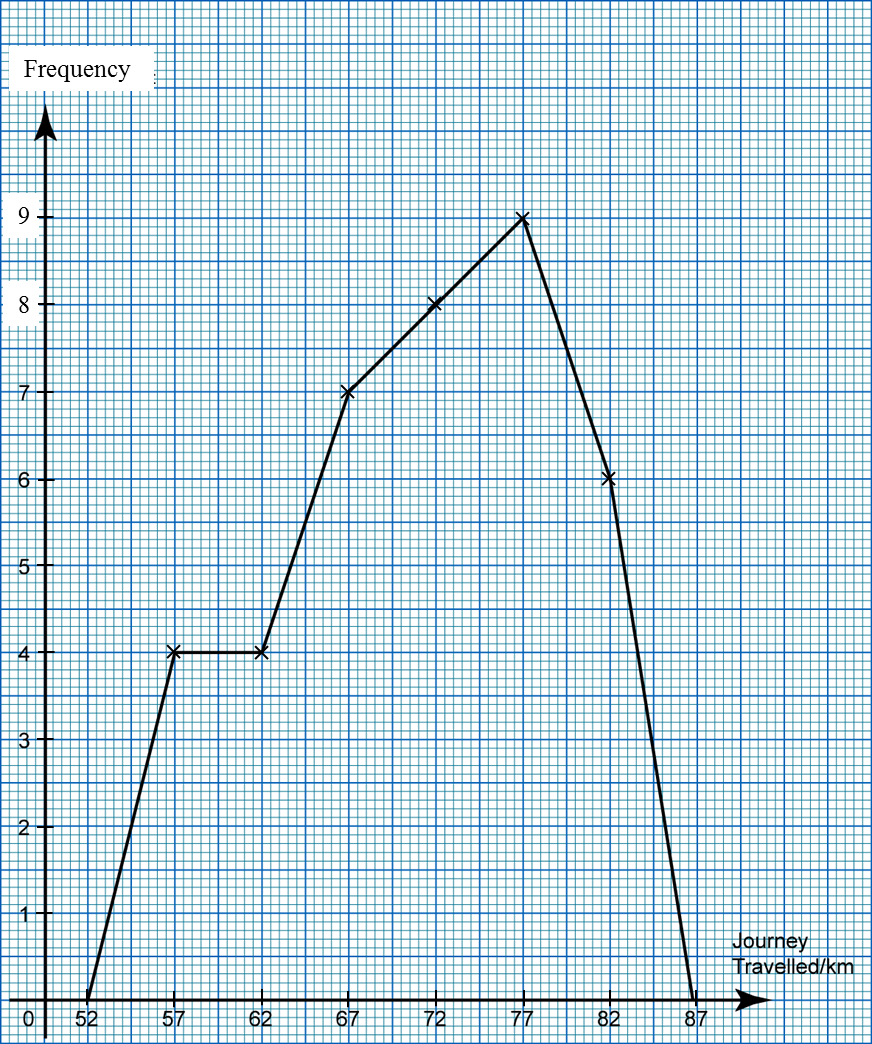# 6.4 Frequency Polygons

6.4 Frequency Polygons

1. A frequency polygon is a line graph that connects the midpoints of each class interval at the top end of each rectangle in a histogram.

2. A frequency polygon can be drawn from a
(a) Histogram,
(b) Frequency table

3. Steps for drawing a frequency polygon:
Step 1: Add a class with 0 frequency before the first class and add also a class with frequency after the last class.
Step 2: Calculate the midpoints or mark the midpoints of the top sides of the rectangular bars including the midpoints of the two additional classes.
Step 3: Joint all the midpoints with straight lines.

Example:
The following frequency table shows the distance travelled by 38 teenagers by motorcycles in one afternoon.

 Journey travelled (km) Frequency 55 – 59 4 60 – 64 4 65 – 69 7 70 – 74 8 75 – 79 9 80 – 84 6
Draw a frequency polygon based on the frequency table.

Solution:

 Journey travelled (km) Frequency Midpoint 50 – 54 0 52 55 – 59 4 57 60 – 64 4 62 65 – 69 7 67 70 – 74 8 72 75 – 79 9 77 80 – 84 6 82 85 – 89 0 87